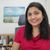# Zonal statistics for multiple rasters

1843
5
11-12-2021 05:27 AM
Status: Open
Labels (1)New Contributor III

Inputs:

• List of rasters
• List of mathematical operations between the rasters
• Zonal raster/polygon
• Statistical attribute (mean, percentile etc...)

For each unique zone in the zonal raster the statistic of the mathemtical function will be calculated.

Example - [raster1,raster2], [+], [zonal raster], [average]

The average of raster1 + raster2 will be calculatedbyHow the zonal statistics tools work—ArcGIS Pro | Documentation

make a multi-dimensional raster from your inputsYes but the aspect of the algebric operation is missing, does it not? Many times I calculate a zonal statistic for the sum or difference of rasters. Using the raster cell iterator helps but having a tool could be awesomebyI suppose, but I usually use numpy for most array (aka, raster) work using the in and out tools provided by arcpy if I need to display the results  (see NumPyArrayToRaster and RasterToNumPyArray)Well any approach would bei OK people like us who could program, but from my experience more and more people without programming expertise come into the GIS world and my suggestion could make life easier for thembyHi Omer, my understanding of this problem is that you want to use the output of a mathematical expression (also known as map algebraic expression in raster analysis) as the value raster input to the Zonal Statistics tool.

Technically, it is a two-steps process. The first step is where the mathematical expression is executed, and the second step is where the zonal operation is executed.

Using ArcPy:

In ArcPy, you can write it using 2 expressions or as one complex expression as follows:

import arcpy

from arcpy.sa import *

in_zone = Raster('zone.tif')

in_ras1 = Raster('ras1.tif')

in_ras2 = Raster('ras2.tif')

# Approach 1: Using two expressions. However, in a standalone script the map algebraic expression will be executed when Zonal Statistics is executed.

out_mapalgebra_ras = in_ras1 + in_ras2

out_zs_ras = ZonalStatistics(in_zone, 'Value', out_mapalgebra_ras)

# Approach 2: Using map algebraic expression as the value raster input

out_zs_complex_ras = ZonalStatistics(in_zone, 'Value', (in_ras1 + in_ras2))

# Approach 3: Creating input raster objects in the complex expression while executing Zonal Statistics

out_zs_complex_ras2 = ZonalStatistics(Raster('zone.tif'), 'Value', (Raster('ras1.tif')+ Raster('ras2.tif')))

Using Geoprocessing tools:

Use the Raster Calculator tool to execute a mathematical expression (also known as map algebraic expression in raster analysis).

Then use the output of Raster Calculator, as the value raster input in the Zonal Statistics tool.

You can also use Model Builder to automate this workflow.

Hope this helps!

Sarmistha